# Test: Calculus- 2

Test Description

## 30 Questions MCQ Test GATE Computer Science Engineering(CSE) 2023 Mock Test Series | Test: Calculus- 2

Test: Calculus- 2 for Computer Science Engineering (CSE) 2023 is part of GATE Computer Science Engineering(CSE) 2023 Mock Test Series preparation. The Test: Calculus- 2 questions and answers have been prepared according to the Computer Science Engineering (CSE) exam syllabus.The Test: Calculus- 2 MCQs are made for Computer Science Engineering (CSE) 2023 Exam. Find important definitions, questions, notes, meanings, examples, exercises, MCQs and online tests for Test: Calculus- 2 below.
Solutions of Test: Calculus- 2 questions in English are available as part of our GATE Computer Science Engineering(CSE) 2023 Mock Test Series for Computer Science Engineering (CSE) & Test: Calculus- 2 solutions in Hindi for GATE Computer Science Engineering(CSE) 2023 Mock Test Series course. Download more important topics, notes, lectures and mock test series for Computer Science Engineering (CSE) Exam by signing up for free. Attempt Test: Calculus- 2 | 30 questions in 90 minutes | Mock test for Computer Science Engineering (CSE) preparation | Free important questions MCQ to study GATE Computer Science Engineering(CSE) 2023 Mock Test Series for Computer Science Engineering (CSE) Exam | Download free PDF with solutions
 1 Crore+ students have signed up on EduRev. Have you?
*Answer can only contain numeric values
Test: Calculus- 2 - Question 1

### Compute the value of: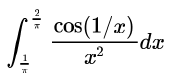Detailed Solution for Test: Calculus- 2 - Question 1

For the integrand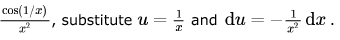This gives a new lower bound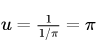and upper bound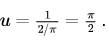Now, our integral becomes: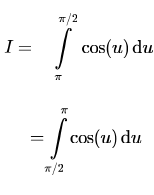Since the antiderivative of cos(u) is sin (u) , applying the fundamental theorem of calculus, we get: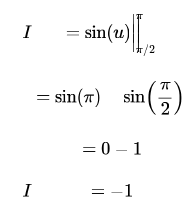Test: Calculus- 2 - Question 2

###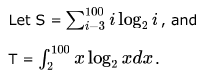Which of the following statements is true?

Detailed Solution for Test: Calculus- 2 - Question 2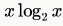is a continuously increasing function, and for a continuously increasing function f(x)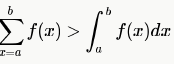But in question, summation of L.H.S. above, a = 3 and in R.H.S, a = 2, so we don't know whether S > T. So we compute some initial values :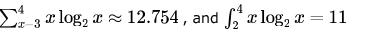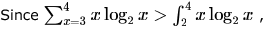and since we already know that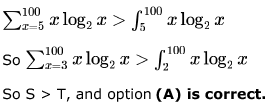Test: Calculus- 2 - Question 3

###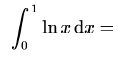Detailed Solution for Test: Calculus- 2 - Question 3

Use Integration by Parts
ln(x) dx
set
u = ln(x),    dv = dx
then we find
du = (1/x) dx,    v = x
substitute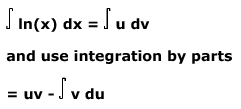substitute u=ln(x), v=x, and du=(1/x)dx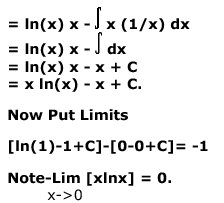*Answer can only contain numeric values
Test: Calculus- 2 - Question 4

(a) Find the points of local maxima and minima, if any, of the following function defined in  0 ≤ x ≤ 6.

x3 - 6x2 + 9x + 15

(b) Integrate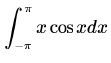Detailed Solution for Test: Calculus- 2 - Question 4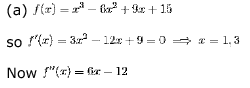f''(1) < 0, so x = 1 is point of local maxima, f''(3) > 0, so x = 3 is point of local minima.
Also the end points 0 and 6 are critical points. 0 is point of local minima, because it is to the left of x = 1 (which is point of maxima). Similarly x = 6 is point of local maxima.

(b) Since xcosx is an odd function, by the properties of definite integration, answer is 0.

*Answer can only contain numeric values
Test: Calculus- 2 - Question 5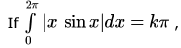then the value of k is equal to ______.

Detailed Solution for Test: Calculus- 2 - Question 5

There is a mod term in the given integral. So, first we have to remove that. We know that x is always positive here and sin x is positive from 0 to π. From π to 2π,  x is positive while sin x changes sign. So, we can write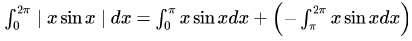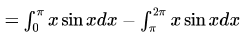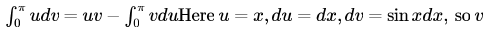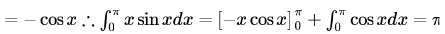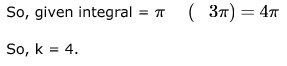Test: Calculus- 2 - Question 6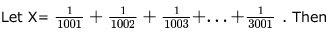Detailed Solution for Test: Calculus- 2 - Question 6

Take the series as 1/x
and use  Riemann integral to evaluate the series
integrate 1/ x dx from x=1001 to 3001 then we get 1.0979 which is in between 1 and 3/2
So C is correct option. 1<X<3/2

Test: Calculus- 2 - Question 7

The value of the integral given below is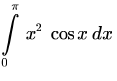Detailed Solution for Test: Calculus- 2 - Question 7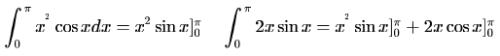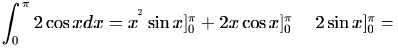Integral of a multiplied by b equals
a multiplied by integral of b
minus
integral of derivative of a multiplied by integral of b

Test: Calculus- 2 - Question 8

The value of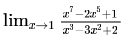Detailed Solution for Test: Calculus- 2 - Question 8

Since substituting x=1 we get 0/0 which is indeterminate
after applying L'H we get ((7x6)-(10x4))/((3x2)-(6x))
now substituting x=1 we get -3/-3
=1

Test: Calculus- 2 - Question 9

What is the value of the following limit?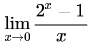Detailed Solution for Test: Calculus- 2 - Question 9

Since we have a 0/0 form, we can apply the L'Hôpital's rule.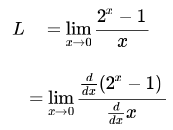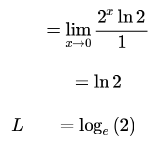Hence, option c is correct.

*Answer can only contain numeric values
Test: Calculus- 2 - Question 10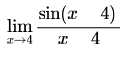=____.

Detailed Solution for Test: Calculus- 2 - Question 10

substitute h=x-4..so it becomes limh->0 (sinh)/h ... which is a standard limit.. Ans would be 1.

Test: Calculus- 2 - Question 11

What is the value of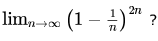Detailed Solution for Test: Calculus- 2 - Question 11

i will solve by two methods
method 1
y=limn->inf (1-1/n)2n
taking log

log y =limn->inf 2n log(1-1/n)        =limn->inf log (1-1/n)/(1/2n)---------------(converted this so as to have form 0/0)
apply l hospital rule
log y=limn->inf (1/1-1/n)1/n2  / (-1/2n2) log y=-2
y=e-2 method two
it  takes 1 to power infinity form

limx->inff(x)g(x)
=elimx->inf(f(x)-1)g(x) (f(x)-1)*g(x)=-1/n*2n=-2 ie -2
constant so we get  final ans e-2

Test: Calculus- 2 - Question 12

The limit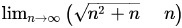equals.

Detailed Solution for Test: Calculus- 2 - Question 12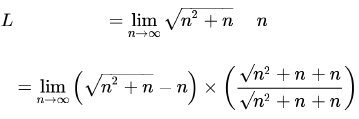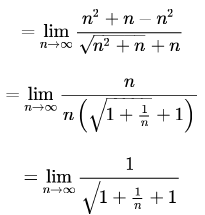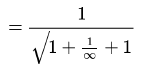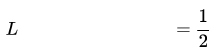*Answer can only contain numeric values
Test: Calculus- 2 - Question 13

In questions 2.1 to 2.10 below, each blank (___) is to be suitably filled in.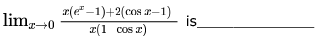Detailed Solution for Test: Calculus- 2 - Question 13

Use LH rule:
First Derivative: [x(ex) + (ex-1) - 2(sinx)]/[xsinx + (1 - cosx)]
Second Derivative: [xex + ex + ex - 2cosx]/[{xcosx + sinx + sinx]
Third Derivative: [xex + ex + ex + ex + 2sinx]/[-xsinx + cosx + cosx + cosx]
Put x = 0: [0+1+1+1+0]/[0+1+1+1] = 3/3 = 1.

Test: Calculus- 2 - Question 14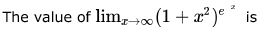Detailed Solution for Test: Calculus- 2 - Question 14

Apply an exponential of a logarithm to the expression.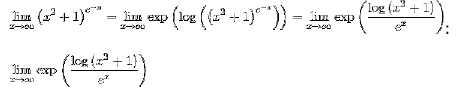Since the exponential function is continuous, we may factor it out of the limit.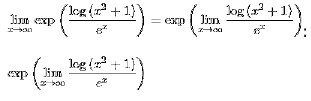The numerator of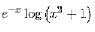grows asymptotically slower than its denominator as x approaches ∞.
Since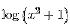grows asymptotically slower than ez as x approaches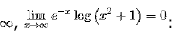e0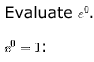Test: Calculus- 2 - Question 15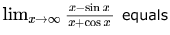Detailed Solution for Test: Calculus- 2 - Question 15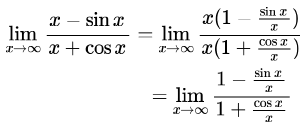now to calculate values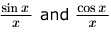we use Squeezing Theorem.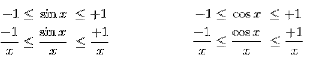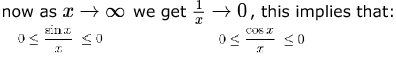Hence,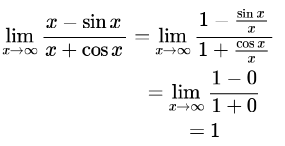Test: Calculus- 2 - Question 16

The limit of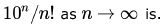Detailed Solution for Test: Calculus- 2 - Question 16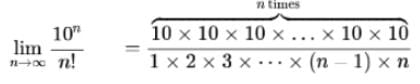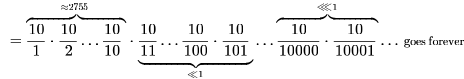Now we can see that after the 10/10 term, all subsequent terms are < 1, and keep decreasing. As we increase the value of n it the product will get close to 0.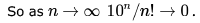Test: Calculus- 2 - Question 17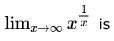Detailed Solution for Test: Calculus- 2 - Question 17

Apply an exponential of a logarithm to the expression.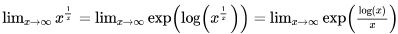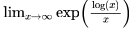Since the exponential function is continuous, we may factor it out of the limit.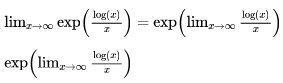Logarithmic functions grow asymptotically slower than polynomials.
since log(x) grows asymptotically slower than the polynomial x as  x approaches ∞,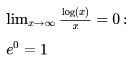Test: Calculus- 2 - Question 18

A point on a curve is said to be an extremum if it is a local minimum or a local maximum. The number of distinct extrema for the curve  is 3x4 + 24x2 + 37 is

Detailed Solution for Test: Calculus- 2 - Question 18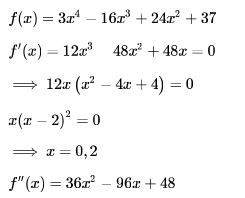at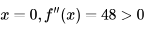it means that x = 0  is local minima.
but at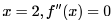so we can't apply second derivative test. So, we can apply first derivative test.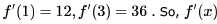is not changing sign on either side of 2. So,x = 2 is neither maxima nor minima.

So, only one extremum i.e. x=0.

*Answer can only contain numeric values
Test: Calculus- 2 - Question 19

Determine the number of positive integers which are not divisible by any of 2, 3 or 5.

Test: Calculus- 2 - Question 20

The maximum possible value of xy2z3 subjected to condition x,y,z>0and x+y+z=3 is

Detailed Solution for Test: Calculus- 2 - Question 20

Given ,              x + y + z = 3
==>    x + (y/2) + (y/2) + (z/3) + (z/3) + (z/3) = 3
Now using A.M. G.M inequality , we have :
[x + (y/2) + (y/2) + (z/3) + (z/3) + (z/3) ] / 6  >=   (x . (y/2) .(y/2) . (z/3) . (z/3) .(z/3))^(1/6)
==>    (x . (y/2) .(y/2) . (z/3) . (z/3) .(z/3))^(1/6)    <=   1/2
==>    (x . (y/2) .(y/2) . (z/3) . (z/3) .(z/3))     <=  1 / 64
==>     x . y2 . z3    <=  108 / 64  =  27 / 16

Test: Calculus- 2 - Question 21

If f(x) is defined as follows, what is the minimum value of f(x) for x ∊ (0, 2] ?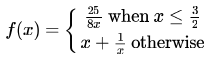Detailed Solution for Test: Calculus- 2 - Question 21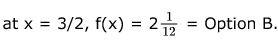Test: Calculus- 2 - Question 22

What is the maximum value of the function   f(x) = 2x2 - 2x + 6 in the interval [0,2] ?

Detailed Solution for Test: Calculus- 2 - Question 22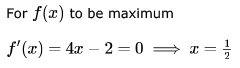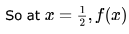is an extremum (either maximum or minimum).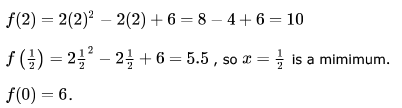So, the maximum value is at x = 2 which is 10 as there are no other extremum for the given function.

Test: Calculus- 2 - Question 23

Consider the problem of maximizing x2 - 2x + 5 such that 0 < x , 2. The value of x at which the maximum is achieved is:

Detailed Solution for Test: Calculus- 2 - Question 23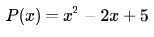Since a polynomial is defined and continuous everywhere, we only need to check the critical point and the boundaries.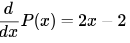Critical point: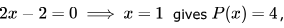which is the minimum.
Boundaries: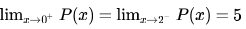Since p(x) increases as x goes farther away from the 1, but
p(x)  is defined on an open interval,
p(x) never attains a maximum!

Hence, e. None of the above is the correct answer.

Test: Calculus- 2 - Question 24

The function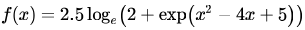attains a minimum at x = ?

Detailed Solution for Test: Calculus- 2 - Question 24

Digvijay is right, f(x) will be minimum at x = 2,
Here is another approach.
Since log and exponent are monotonically increasing functions, the problem of minimizing f(x) can be reduced to just minimizing the quadratic expression
x2 - 4x + 5,
this  quadratic expression can be written as (x2 - 4x + 4) + 1 which is equal to
(x - 2)2 + 1.
now since (x - 2)^2 can not be less than 0, so (x - 2)^2 + 1 can not be less than 1.
Also (x - 2)2 + 1 will be at its minimum value (= 1), when x = 2.
so value of f(x) will be minimum at x = 2.

Test: Calculus- 2 - Question 25

Suppose that f(x) is a continuous function such that  0.4 ≤ f(x) ≤ 0.6 for 0 ≤ x ≤ 1.  for  Which of the following is always true?

Detailed Solution for Test: Calculus- 2 - Question 25

This is a repeating question on continuity. Let me solve it a non-standard way -- which should be useful in GATE.
From the question f is a function mapping the set of real (or rational) numbers between [0,1] to [0.4,0.6]. So, clearly the co-domain here is smaller than the domain set. The function is not given as onto and so, there is no requirement that all elements in co-domain set be mapped to by the domain set. We are half done now. Lets see the options:

A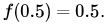False, as we can have f(0.5) = 0.4, continuity does not imply anything other than all points being mapped being continuous.
C. Again false, we can have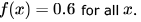for all .
D. False, same reason as for A.

D. False, same reason as for A.
Only B option left- which needs to be proved as correct now since we also have E option. We know that for a function all elements in domain set must have a mapping. All these can map to either 1 or more elements but at least one element must be there in the range set. i.e., f(x) = y is true for some y which is in [0.4,0.6]. In the minimal case this is a single element say c. Now for x = 1/0.8, option B is true. In the other case, say the minimal value of f(x) = a and the maximum value be f(x) = b. Now,

as per Intermediate Value theorem between  and  are also in the range set as f is continuous. Now, we need to consider x in the range [0.5, 0.75] as then only f(x) can be 0.8x and be in [0.4,0.6]. In our case we have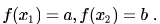Lets assume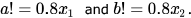Now, for all other points in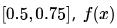must be between a and b and all points between a and b must be mapped by some x.

Moreover,for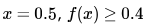aand for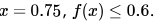So, if we plot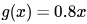this line should cross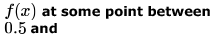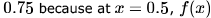must be above or equal to the line 0.8x (shown below) and for x = 0.75 it must be belowor equal which means an intersection must be there.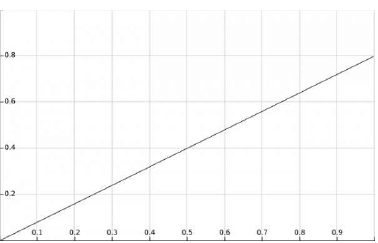This shows there exist some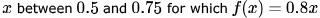a stronger case than option B. So, B option is true. Now please try for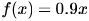and see if it is true.

Test: Calculus- 2 - Question 26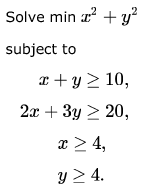Detailed Solution for Test: Calculus- 2 - Question 26

x>=4 and y>=4 , So we can take both x =5 & y = 5
x+y >= 10 => Satisfied , 5+5 = 10
2x + 3y >= 20. Satisfied.
This is infact minimum value.
Other options =>
4,4 => x+y constraint fail
4,5 => x+y fail
6,4 => Still giving 52 as sum which is more than 50 !,  This can not  be answer.
7,3 => 49+9 > 58 > 50.

Test: Calculus- 2 - Question 27

The minimum of the function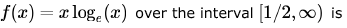Detailed Solution for Test: Calculus- 2 - Question 27

Minimum value of function occurs at end points or critical points
f'(x)=1+logx
Equate it to 0
x=1/e
f''(x)=1/x
Put x=1/e f''(x)=e so minima at 1/e But 1/e=0.36
But x∈[1/2,infinity)
So min occurs at 1/2
So min value=1/2 log 1/2
So ans is c

Test: Calculus- 2 - Question 28

Consider the differential equation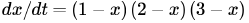. Which of its equilibria is unstable?

Detailed Solution for Test: Calculus- 2 - Question 28

For unstable equilibrium point, dx/dt >0
At x = 0, dx/dt =  (1-0)(2-0)(3-0) = 6 > 0
Hence x = 0 is point of unstable equilibrium.
We can understand equilibrium in terms of radioactive decay.
Let dN/dt = -KN ;K>0 its significance is that an element is loosing energy so it is getting stability because we know more energy an element gets,more de-stability it gains and vice versa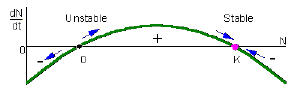Test: Calculus- 2 - Question 29

Consider the function f(x) = sin x in the interval x = [π/4 , 7π /4]. The number and location(s) of the local minima of this function are

Detailed Solution for Test: Calculus- 2 - Question 29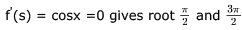which lie between given domain in question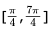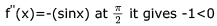it means it is local maxima and at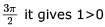which is local minima and since it at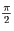is local maxima so before it graph is strictly increasing so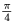is also local minima
so there is two local minima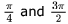Sine function increases till π/2 and so for the considered interval π/4 would be a local minimum. From π/2, value of sine keeps on decresing till 3π/2 and hence 3π/2 would be another local minima. So, (D) is the correct answer here.

Test: Calculus- 2 - Question 30

The equation 7x7 + 14x6 + 12 x5 + 3x4 + 12x3+ 10x2 + 5x+ 7 = 0 has

Detailed Solution for Test: Calculus- 2 - Question 30

Since the polynomial has highest degree 7. So there are 7 roots possible for it
now suppose if an imaginary number a+bi is also root of this polynomial then a-bi will also be the root of this polynomial
That means there must be even number of complex root possible becoz they occur in pair.
Now we will solve this question option wise
A) All complex root
This is not possible. The polynomial has 7 roots and as I mention a polynomial should have even number of complex root and  7 is not even. So this option is wrong
B) At least one real root
This is possible. Since polynomial has 7 roots and only even number of complex root is possible, that means this polynomial has max 6 complex roots and Hence minimum one real root. So this option is correct
C) Four pairs of imaginary roots 4 pair means 8 complex root. But this polynomial can have atmost 7 roots. So this option is also wrong

## GATE Computer Science Engineering(CSE) 2023 Mock Test Series

150 docs|215 tests
 Use Code STAYHOME200 and get INR 200 additional OFF Use Coupon Code
Information about Test: Calculus- 2 Page
In this test you can find the Exam questions for Test: Calculus- 2 solved & explained in the simplest way possible. Besides giving Questions and answers for Test: Calculus- 2, EduRev gives you an ample number of Online tests for practice

## GATE Computer Science Engineering(CSE) 2023 Mock Test Series

150 docs|215 tests